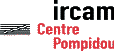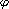Serveur © IRCAM - CENTRE POMPIDOU 1996-2005.Tous droits réservés pour tous pays. All rights reserved.

# Perceptual characterisation of vehicules noises

Patrick Susini, Stephen Mcadams et Suzanne Winsberg

EEA Symposium: "Psychoacoustic in Industry and Universities", Janvier 1997

Version Française

## Abstract

To improve sound quality in cars, a preference map was created on the basis of several acoustic parameters relevant to auditory perception. A multidimensional scaling technique (CLASCAL) was used to reveal common perceptual dimensions shared by sets of car sounds, perceptual features specific to each sound, and the different subject classes among listeners. The listeners' task was to judge the degree of dissimilarity of all pairs of car sounds on a continuous scale. The analysis gives a perceptual spatial representation of the sounds. From this analysis, acoustic and auditory modelling analyses indicated that a number of stimulus parameters were strongly correlated with different perceptual dimensions and, where possible, with the specific features. A further experiment investigated the probability of one sound being preferred to another. An analysis of the data allowed a projection of the structure of listeners' preferences onto the physical parameter space underlying the previously determined multidimensional perceptual space. In many cases, it was found that the physical parameters having the most effect on the listeners' preferences were dependent on the set of stimuli being compared. Furthermore, when one stimulus parameter was kept constant across trials, this altered the effects of other parameters on the listeners' preferences.

## 1. Introduction

With the aim of improving the sound quality of car interiors, a psychoacoustic study was performed at IRCAM in collaboration with two industrial partners, Renault and PSA Peugeot Citroën. This study sought to determine experimentally the perceptual structures underlying the comparison of car sounds. Sixteen sounds were recorded with artificial heads on a test track with a stable motor regime and were then presented binaurally to subjects under controlled listening conditions. The first step consisted in determining the perceptual attributes common to a group of subjects that judged the sounds with respect to one another. A mutidimensional analysis with the CLASCAL model (Winsberg & De Soete, 1993a) yielded a clear graphic representation that revealed the perceptual structure underlying the judgments in terms of continuous dimensions shared by all the stimuli and of characteristics specific to each stimulus ("specificities"). The CLASCAL model also allows one to account for different judgment strategies used by latent classes of subjects by estimating the perceptual weights assigned to each dimension and the set of specificities by each class. A subsequent phase of acoustic analyses sought to determine the signal parameters that were correlated with the position of each sound sample along each perceptual dimension. In a final stage, the degree of judged preference associated with each sound as a function of the perceptually significant parameters was estimated.

The perceptual representation model thus obtained provides constructors with a tool with which the sound space of vehicle interiors can be improved according to criteria associated with auditory quality.

## 2. Dissimilarity study

### 2.1. Method

2.1.1. Subjets and stimuli
In the dissimilarity study, 30 subjects aged from 25 to 45 years were recruited. In order to study the influence of certain parameters on the perceptual representation of car noises, four groups of stimuli were used in all of the experiments. The cars were the same for each of the groups. Two motor regimes were used (fixed motor and ground speed combinations). In addition, for each regime, one acoustic parameter1, was variable from one stimulus to another in a first experiment series. In a second series, this parameter was equalized over the set of stimuli. The composition of the four groups of sounds is summarized in Table I.

 Regime R1 Regime R2 Parameter1 variable Group 1 Group 2 Parameter1 constant Group 3 Group 4

Table I : Composition of four groups of stimuli used.

2.1.4. Procedure
The experiment was conducted in four sessions with one session for each stimulus group. All 120 pairs of different sounds for the 16 cars were presented. At the beginning of the session, the subjects listened to all samples for a given regime in a random order to get a sense of the range of possible variation. Then 15 practice trials were presented to familiarize the subject with the task. In each trial, the order of presentation of the pair of samples was chosen randomly. A 1 s silence separated the two sounds which each lasted about 5 s. The subject entered the dissimilarity judgment by moving a slider on the computer screen that represented a continuous scale from very similar at the left (coded 0) to very dissimilar at the right (coded 1). Each sound pair could be replayed at will until the subject was satisfied with the judgment. Once the judgment was entered, the next trial was automatically presented.

### 2.2. Results

A Monte Carlo simulation was performed to determine the number of latent classes that existed in the population of subjects tested. In our case, a single class was sufficient to account for each stimulus group. Analyses for from one to six dimensions without specificities and for from one to five dimensions with specificities was performed for a single class of subjects using the CLASCAL model. The information criterion BIC indicated that the best solution had three dimensions with specificities for Group 3 and two dimensions with specificities for the other stimulus groups. A graphic representation of the solution for Group 3 stimuli is shown in Figure 1.

## 3. Physical parameters underlying the perceptual space

### 3.1. Acoustic analyses

A set of acoustic and psychoacoustic parameters (1,2,3,4 and5) was chosen by way of an empirical loop that consisted in looking for parameters that were well correlated with the coordinates of stimuli along a given dimension of the selected solution. Given the quasi-stationary nature of the signals, the signal analyses were based on the spectral analysis method of Welch.

## 3.2. Results

 Groupe 1 Groupe 2 Param. Dim. 1 Dim. 2 Dim. 1 Dim. 21 -0.92* -0.06 -0.91* 0.282 0.09 0.80* - -3 - - 0.43 -0.88*

 Groupe 3 Groupe 4 Param. Dim. 1 Dim. 2 Dim. 3 Dim. 1 Dim. 22 0.35 -0.7* -0.14 -0.93* -0.293 - - - 0.51+ 0.86*4 -0.81* 0.32 -0.33 - -5 -0.32 0.00 -0.83* - -

Table II. Correlation coefficients between perceptual coordinates and "objective" parameters. The probability p that the two measures are independent is indicated for p < 0.01 (*) and for p < 0.05 (+).

In Table II, the correlation coefficients between the perceptual dimensions and the acoustic or psychoacoustic parameters are shown for each stimulus group.

## 4. Preference study

### 4.1. Method

4.1.1. Subjets and stimuli
In this study, 60 subjects aged from 25 to 45 years were recruited. The stimuli were the same as those used in the dissimilarity study.

4.1.2. Procedure
All pairs of different samples (in both orders) were presented in random order. In each trial the subject heard the pair of samples once and was required to chose which sound was preferred.

### 4.2. Preference analysis

For the set of subjects, a single triangular matrix without diagonal was computed. An entry in the matrix corresponded to the probability that one sample was preferred over the other. This probability is defined in the model as a function of the difference in "utility" of the objective factors that characterize the samples (Winsberg & De Soete, 1993b). In the analysis that follows, these factors are the acoustic and psychoacoustic parameters that were shown to have good correlations with the perceptual dimensions revealed in the dissimilarity study. Since the perceptual spaces had two or three common dimensions, we tested different pairs of corresponding parameters for two-dimensional preference spaces. The analysis program searches for a function that transforms the value of the objective parameter into a utility value that depends on the empirical preference probability. This dependence is expressed in the following way:
pi,j =(ui - uj), (1)

where pi,j is the probability that sample i will be preferred over sample j,is the cumulative normal function, and ui is the utility of sample i. As the difference in utility between the two samples increases and is positive, the probability that sample i will be preferred over sample j increases. The utility value is not identical to the preference probability, but has a montonic relation to it.

### 4.3. Results

A graphic representation of the results allows a visualisation of the evolution of utility of one stimulus in relation to another as a function of the objective parameters determined in the dissimilarity analysis. In the simple case where the sound pressure level would be a parameter revealed by such an approach, its utility function may resemble that shown in Figure 2.

Figure 1. Perceptual space of the stimuli from Group 3.

Figure 2. Evolution of the utility of sound level.

## 5. Conclusion

In the first series of experiments performed with the parameter1 not being equalised, this parameter clearly dominated the preference judgments for the two regimes, in spite of other parameters having equivalent degree of effect on dissimilarity judgments. The secondary parameters varied with regime and had a lesser importance for preference judgments. This indicates that equal sensitivity in the case of dissimilarity judgments does not guarantee equal effect in terms of preference. When the samples were equalized for parameter1, other factors that contribute to preference emerge. In this case, no single parameter dominated preference judgments across the two regimes. In general, the perceptual salience of the dimensions was not the same for the two regimes, which indicates that the relative importance of perceptual cues evolves with regime and comparison context.

*This paper is based on an article submitted to the 4th French Acoustics Congress, Marseille, April 1997, to appear in Journal de Physique (Susini, McAdams & Winsberg, 1997).

## References

Susini, P., McAdams, S. & Winsberg, S. (1997) Caractérisation perceptive des bruits de véhicules. Journal de Physique (sous presse).

____________________________
Server © IRCAM-CGP, 1996-2008 - file updated on .

____________________________
Serveur © IRCAM-CGP, 1996-2008 - document mis à jour le .